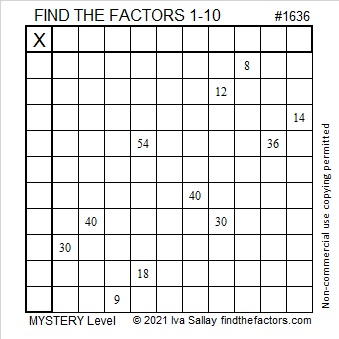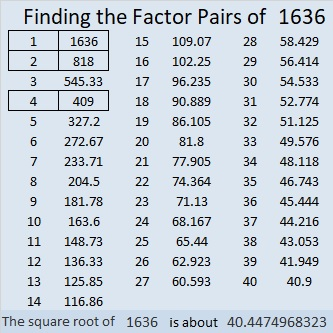# 1636 A Tricky Mystery Puzzle

Contents

### Today’s Puzzle:

It took me a while to figure out the logic of this puzzle so don’t get discouraged if you find it a bit tricky, too.If its logic eludes you, this short video I posted on Twitter should help.

### Factors of 1636:

• 1636 is a composite number.
• Prime factorization: 1636 = 2 × 2 × 409, which can be written 1636 = 2² × 409.
• 1636 has at least one exponent greater than 1 in its prime factorization so √1636 can be simplified. Taking the factor pair from the factor pair table below with the largest square number factor, we get √1636 = (√4)(√409) = 2√409.
• The exponents in the prime factorization are 2 and 1. Adding one to each exponent and multiplying we get (2 + 1)(1 + 1) = 3 × 2 = 6. Therefore 1636 has exactly 6 factors.
• The factors of 1636 are outlined with their factor pair partners in the graphic below.### More about the Number 1636:

1636 is the sum of two squares:
40² + 6² = 1636.

1636 is the hypotenuse of a Pythagorean triple:
480-1564-1636, calculated from 2(40)(6), 40² – 6², 40² + 6².
It is also 4 times (120-391-409).

1636⁴ = 7,163,630,838,016. Thank you OEIS.org for that fun fact!

This site uses Akismet to reduce spam. Learn how your comment data is processed.